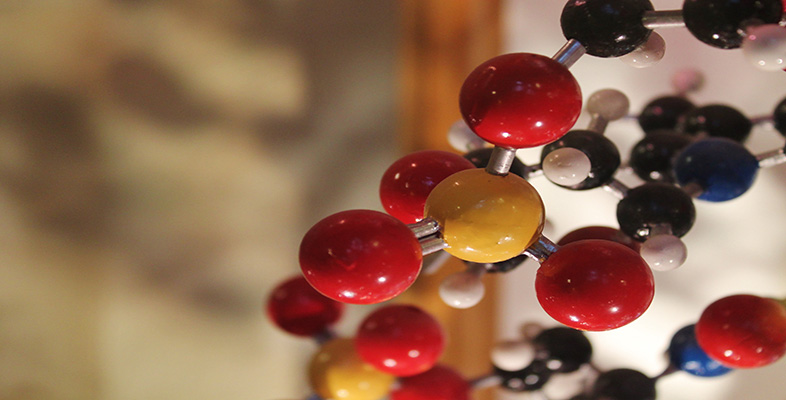Basic science: understanding numbers

Start this free course now. Just create an account and sign in. Enrol and complete the course for a free statement of participation or digital badge if available.

Free course

# 3.2.1 Rounding to decimal places

Often when you use your calculator to do calculations, you will obtain an answer with many numbers following the decimal point. The numbers after the decimal point are referred to as decimal places. For example, consider the number 155.76403. There are five numbers after the decimal point, so you would say the number is given to ‘five decimal places’. The number of decimal places you see may depend on your calculator, although usually calculators can show at least seven decimal places.

Although it may be tempting to give this entire number as your answer, it may not reflect its real accuracy, so the number needs to be rounded. This also makes the number easier to read, write and understand.

When rounding a number, you need to decide to how many decimal places you are confident in your answer. If you are confident of the answer to two decimal places, you would round to 155.76. Before you discard the rest of the numbers, you need to work out whether to round the final digit up or down. If the first digit that you intend to discard is a 0, 1, 2, 3 or 4 then you can go ahead and discard them. If the first digit that you intend to discard is a 5, 6, 7, 8 or 9, then you have to increase your final digit by one. For example, if the number 155.76403 is to be rounded to one decimal place, you must look at the second decimal place, which is a six, and then round the first decimal place up by one, so the answer is 155.8

Earlier in this week, you were introduced to an experiment to find the water content of different fruits. The initial weight of each fruit was measured in grams and presented to three decimal places with the watermelon weighing 160.156 grams, the cucumber weighing 127.751 grams and the peach weighing 64.375 grams.

If you were working with these fruits in a recipe, for example, it might be more appropriate to report the weights as whole numbers, with no decimal places. So, in this example, the first decimal place immediately follows our chosen cut off, and is the value we use to round our answer. For the watermelon it’s a 1, for the cucumber it’s a 7 and for the peach it’s a 3.

Only the cucumber has a first decimal place with a value over 5, so the last remaining digit needs to be rounded up. As for the watermelon and the peach, their numbers are less than 5, so they aren’t rounded up. The weights of these three fruits can therefore be written as 160, 128 and 64 grams.

Last week, we calculated that 361,648,138 km2 of the Earth’s surface is covered by oceans. To simplify things we rounded this number to 361,650,000. Hopefully you can now understand how we obtained this rounded number. The next section covers rounding to significant figures, which is more commonly seen in science.